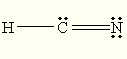# Which one of the following is expected to exhibit resonance? (A) CO_2 (B) NH_4^(+) (C) HCN (D) NO_2""^(-)

Jun 28, 2015

The expected answer is probably D) ${\text{NO}}_{2}^{-}$.

#### Explanation:

Both ${\text{CO}}_{2}$ and $\text{HCN}$ also exhibit resonance, but they have only one major contributor. ${\text{CO}}_{2}$ has two major contributors.

To determine if resonance is possible, you draw the Lewis structure and then see if you can put the electrons in different locations.

A) ${\text{CO}}_{2}$

The Lewis structure for ${\text{CO}}_{2}$ isThere are two other resonance contributors, but they involve charge separation, so they are minor contributors.B) ${\text{NH}}_{4}^{+}$

The Lewis structure of ${\text{NH}}_{4}^{+}$isThis is the only Lewis structure — no resonance.

C) $\text{HCN}$

The Lewis structure of $\text{HCN}$ isYou can draw a structure with a $\text{C=N}$ bond, but it puts formal charges on $\text{C}$ and $\text{N}$, so it is a minor contributor.D) ${\text{NO}}_{2}^{-}$

${\text{NO}}_{2}^{-}$ has two equivalent Lewis structures. They are both major resonance contributors.